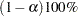MODEL Statement
MODEL dependent = <effects> </ options> ;

The MODEL statement gives the dependent variables and independent effects. If you specify more than one dependent variable, a separate analysis is performed for each one. The independent effects are limited to main effects, interactions, and nested effects; no continuous effects are allowed. All independent effects must be composed of effects that appear in the CLASS statement. Effects are specified in the VARCOMP procedure in the same way as described for the ANOVA procedure. Only one MODEL statement is allowed.

The following options are available in the MODEL statement.

FIXED=n

specifies that the first n effects in the MODEL statement are fixed effects. The remaining effects are assumed to be random. By default, PROC VARCOMP assumes that all effects are random in the model. Keep in mind that if you use bar notation and, for example, specify Y=A|B / FIXED=2, then A*B is considered a random effect.

CL=
CL=MLS
CL=GCL<(options)>

specifies that confidence limits for all of the parameters of interest be computed and displayed. It also optionally specifies the method to use for computing the confidence limits. There are two methods: the modified large-sample (MLS) method and the generalized confidence limits (GCL) method. The default method is MLS. For more information about these two methods, see the section Confidence Limits.

You can specify the following options in parentheses after CL=GCL.
NSAMPLE=n

specifies the sample size for generalized pivot quantities (GPQ) sampling. The default value is 12,605.

EPSILON=number

specifies a small positive value used in some GPQ computations. The default value is 0.001.

The CL option applies only to balanced one-way or two-way designs for METHOD=TYPE1 or GRR.

ALPHA=specifies the level of significancefortwo-sided confidence limits. The value ofmust be between 0 and 1. By default,is equal to 0.05.